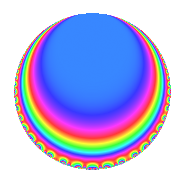# Properties

 Label 800.1.eLevel 800 Weight 1 Character orbit e Rep. character $$\chi_{800}(399,\cdot)$$ Character field $$\Q$$ Dimension 2 Newforms 1 Sturm bound 120 Trace bound 0

# Related objects

## Defining parameters

 Level: $$N$$ = $$800 = 2^{5} \cdot 5^{2}$$ Weight: $$k$$ = $$1$$ Character orbit: $$[\chi]$$ = 800.e (of order $$2$$ and degree $$1$$) Character conductor: $$\operatorname{cond}(\chi)$$ = $$40$$ Character field: $$\Q$$ Newforms: $$1$$ Sturm bound: $$120$$ Trace bound: $$0$$

## Dimensions

The following table gives the dimensions of various subspaces of $$M_{1}(800, [\chi])$$.

Total New Old
Modular forms 32 4 28
Cusp forms 8 2 6
Eisenstein series 24 2 22

The following table gives the dimensions of subspaces with specified projective image type.

$$D_n$$ $$A_4$$ $$S_4$$ $$A_5$$
Dimension 2 0 0 0

## Trace form

 $$2q + O(q^{10})$$ $$2q + 2q^{11} - 2q^{19} - 2q^{41} - 2q^{49} - 2q^{51} + 4q^{59} - 2q^{81} + 2q^{89} + O(q^{100})$$

## Decomposition of $$S_{1}^{\mathrm{new}}(800, [\chi])$$ into irreducible Hecke orbits

Label Dim. $$A$$ Field Image CM RM Traces $q$-expansion
$$a_2$$ $$a_3$$ $$a_5$$ $$a_7$$
800.1.e.a $$2$$ $$0.399$$ $$\Q(\sqrt{-1})$$ $$D_{3}$$ $$\Q(\sqrt{-2})$$ None $$0$$ $$0$$ $$0$$ $$0$$ $$q-iq^{3}+q^{11}-iq^{17}-q^{19}-iq^{27}+\cdots$$

## Decomposition of $$S_{1}^{\mathrm{old}}(800, [\chi])$$ into lower level spaces

$$S_{1}^{\mathrm{old}}(800, [\chi]) \cong$$ $$S_{1}^{\mathrm{new}}(200, [\chi])$$$$^{\oplus 3}$$